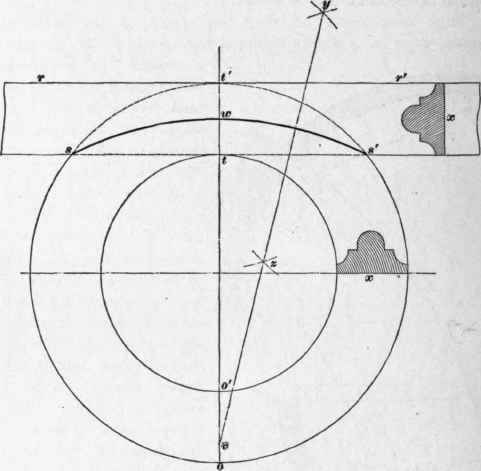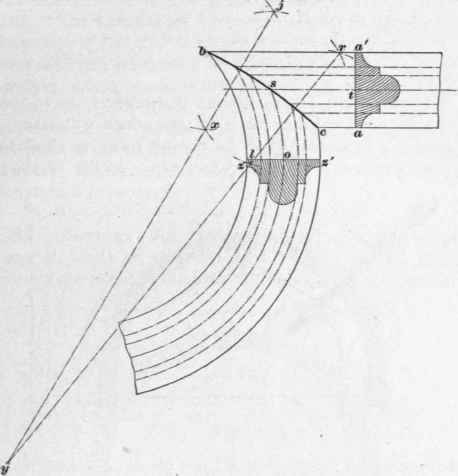To insure a better understanding- of this work, a perspective drawing- of the conelike solid, the surface of which corresponds to the contour of the inside of this arched opening, is shown in Fig. 47. At the interior, the opening is circular, as shown at o' u o, and, the top uj being level, the height jl on the exterior is the same as the height ku on the interior; but the sides of the opening splay towards the outside, and the width on the exterior a' a is greater than the width on the inside o' o, so that the exterior elevation of the opening a' ja is elliptical. The other letters in Fig. 47 refer to the same points as similar letters in Fig. 46.

81. A plan of a window or door opening in a concave circular wall, with the jambs splayed, the soffit a semicircle on one side of the wall, and the other side a semicllipse, the crown of the soffit being level, is shown in Fig. 48. Let B be the circular wall, and bb' dd' the opening and splay of the jambs; continue the splayed face of jambs b' d' and bd to g; from g through the center of opening draw the center line gy indefinitely; from /, the intersection of this center line with the line dd', describe the semicircle d'p d, and divide it into any number of equal parts, through each of which, as i, n, etc., let fall perpendiculars, ic, na', etc., to d'd; from g draw lines through c and a' to e' and l; perpendicular to b' b draw e' m and l m' equal respectively to c i and a' n; make q y equal to f p, and then trace the exterior semi-ellipse b m m'y b'.

To find the length and shape of soffit, draw gs at right angles to bg; make gk, gz, etc. equal to ci, a' n, etc., and with s as a center, describe the arc gw x of indefinite length. With go as a radius, and k as a center, describe an arc, and with di as a radius, and d as a center, describe a second arc cutting the first at c" through c' draw ks". Next with ga as a radius, and z as a center, describe an arc, and with i n as a radius, and c' as a center, describe a second arc intersecting the first at n'; with g h as a radius, and s as a center, describe an arc at v; with up as a radius, and n' as a center, describe an intersecting arc at v, and through s and v draw sv' indefinitely. Make c's" n' t" and vv' equal to oe, al', and hj, etc., respectively. Make w x equal to wg; connect sx; make so"x' equal to s z k; from s as a center, with any convenient radius, describe a measuring arc rp' u, make p' u equal to p'r; through u draw xb". Lay off uj, ub", r' z', r'g' u'h', and u' f equal respectively to rd, rb, k'c', k's", o' n', and o' t"; through the points d, c', n', v, h' z',f trace a curve, which will be the convex edge of the soffit; and through the points b, s", t", v', f', g', b" trace a second curve, which will be the concave edge. The final directions and explanations given in connection with Figs. 46 and 47 apply equally in this case.Fig. 48

82. A circular seat with inclined back is shown at a b d c in Fig. 49. To find the length and shape of the back, let a b be an end section of the seat and eh an end section of the back; the upper line b a of the seat is continued to /, the distance bf being equal to the radius of the back of the seat; and fd is drawn at right angles to fb. Now, withf as a center, and with a radius fb, describe the quarter circle bd, and with a radius fa equal to the radius of the front edge of the seat, describe the quarter circle a c. Then abdc will be the plan of the seat. To unfold the length and shape of the back, prolong h e indefinitely, and prolong df until it intersects he at m; with m as a center describe the arcs h k, b l, and ep. Divide the quarter circle b d into any number of equal parts, say five; lay off these five parts from b to l, and from in draw m k through l; then the length and shape of the back will be p k h c.Fig. 49.

This back must be bent, by some of the methods given, over a suitably constructed center. To prepare a center as required for bending the unfolded back, lay out cmh, Fig. 50, to correspond in dimensions with c rn h in Fig. 49. Draw vv' at a distance from c h equal to the thickness of the material of which the staves, or lagging, are to be made. Then with n as' a center, and with n v as a radius, draw the arc vg, which will be the curve to which the upper center pieces t are to be sawed. The lower center pieces /' are sawed to the curve described with a radius rv'. The edges of the ribs must be beveled to suit the angles shown at v and v', and the lagging applied to these two center pieces brings the exterior curve up to the required form, around which the back of the seat may be bent.Fig. 50.

83. To miter a straight and circular molding, the straight molding to be tangent to the circular one: Let oo'tt', in Fig. 51, be the circular molding, and x a cross-section of it; let ss' rr' he a. straight molding, with the same cross-section x. Bisect t t' at w, and with s' and w as centers, describe the intersecting arcs through y and z; join y and z and produce the line to intersect of in v. Now from v as a center, describe the arc s w s', which will be the miter line required.Fig. 51.

84. To miter a straight and circular molding intersecting at an acute angle: Let b a' a c, Fig. 52, be the straight molding, and a a' the cross-section; let sz' cb be the circular molding, and zz' its cross-section; through the straight molding draw a center line t s; through the circular molding describe a center line o s. With c and s as centers, describe the intersecting arcs r and l and through r and l, draw the line ry of indefinite length. With s and b as centers, describe the intersecting arcs at j and x, and through these points draw the line jy, cutting the line r l in the point y; with y as a center, describe the curve b sc, which will be the miter line required. These miter lines on circular moldings are called hunting miters.Fig. 52.